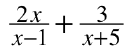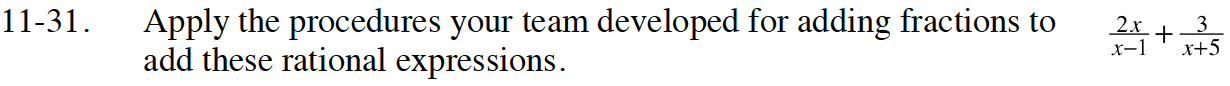Home > INT3 > Chapter 11 > Lesson 11.1.3 > Problem11-31

11-31.
1. Apply the procedures your team developed for adding fractions to add these rational expressions. Homework Help ✎

2.The common denominator is (x − 1)(x + 5).

$\frac{2x^2+13x-3}{(x-1)(x+5)}$